# Chinese Alt Text Writing Based on Deep Learning

Chinese Alt Text Writing Based on Deep Learning

Jinbao Xie Ruitong Li Shiwei Lv Yujing Wang Qiangyan Wang Yury I. Vorotnitsky

School of Electrical and Electronic Engineering, Harbin University of Science and Technology, Harbin 150080, China

Department of Telecommunications and Information Technology, Belarusian State University, Minsk 220030, Belarusian

Corresponding Author Email:
jbxpost@hrbust.edu.cn
Page:
161-170
|
DOI:
https://doi.org/10.18280/ts.360206
25 January 2019
|
Accepted:
3 April 2019
|
Published:
5 July 2019
| Citation

OPEN ACCESS

Abstract:

To generate coherent and readable Chinese image caption, this paper designs an Chinese image captioning model based on Inception-ResNet-v2, a deep convolutional neural network (DCNN) based on residual blocks, and the double-layer gated recurrent unit (GRU) network. The proposed model extracts the features from the original image with the Inception-ResNet-v2. To overcome the stochasticity of random text encoding, the neural network modelling was performed to create word embedding features for sparse word codes. Next, the extracted deeply convoluted image features were mapped to the word embedding feature space. Finally, the double-layer GRU network was trained with the image features and word embedding features, yielding the Chinese image captioning model. The proposed model was proved through experiment as capable of generating Chinese text for images. In addition, our model performed excellently in the objective evaluation with indices like Perplexity, BLEU and ROUGE-L. Specifically, the Perplexity score of our model was 4.922, the BLEU-1, BLEU-2, BLEU-3 and BLEU-4 results were 0.674, 0.533, 0.416 and 0.330, respectively, and the ROUGE-L was 0.635. All of these were better than the results of the other models like the natural image captioning (NIC) model.

Keywords:

Chinese image captioning, deep convolutional neural network (DCNN), feature extraction, gated recurrent unit (GRU) network

1. Introduction

The concept of alt text was first proposed by Farhadi et al. , with the aim to facilitate the grasp of image content despite the complexity of visual scenes. The alt text can greatly facilitate the organization of image data, as well as the mining of large amounts of data through information retrieval. The writing techniques of English alt text are relatively mature, such as the depth semantic alignment model , the guiding the long-short term memory model (gLSTM)  and the natural image captioning (NIC) model . By contrast, the research on Chinese alt text is far less advanced, due to the difficulty in encoding Chinese sentences. After all, a Chinese sentence is much more ambiguous in semantics, and harder to segment into words than an English one. The writing of an effective Chinese alt text requires the integration between computer vision and natural language processing. The alt text should include a tag about the image category, and a highly-readable sentence that sums up the image content .

Taking the 2017 AI Challenger Competition image caption dataset as the training data, this paper designs a novel Chinese alt text writing model based on the encoding and decoding ideas of the NIC model. The model encodes and decodes RGB images and generates Chinese sentences, using the deep convolutional neural network (DCNN) and double-layer gated recurrent unit (GRU) network. To overcome the sparsity of word codes, the sparse word vectors were modelled and the word embedding features were extracted by the neural network language model (NNLM), thereby reducing the dimensionality without sacrificing the semantic relations among sentences.

2. Basic Principle and Implementation Method

2.1 Extraction of image features

The performance of an alt text writing model can be determined by the expressiveness of the extracted image features. With the emergence of AlexNet , the DCNN has attracted much attention for its excellence in image feature extraction and image classification . In this paper, the DCNN model is selected to extract image feature descriptors, considering its advantages over the traditional manual feature extraction: (1) The kernel parameters of the DCNN are self-learned, eliminating human interference; (2) With many convolution kernels and multiple layers, the DCNN can learn a huge number of features and extract features on high levels; In this way, the deep features obtained through integration will be more expressive and richer in semantic information.

Nevertheless, the numerous network layers may cause problems like vanishing gradient and exploding gradient. In this case, the DCNN converges slowly and even does not converge during training. The vanishing gradient problem can be solved simply by regularizing the initial terms, but this solution will lead to network degradation. The ResNet  offers a better solution called long skip connections, which activates the network from a certain layer, and provides immediate feedbacks to the deeper layers. The basic units of the solution are residual blocks. As shown in Figure 1, a typical residual block involves the summation of x with the residual function F(x) on two weight layers beyond the original network, followed by the nonlinear activation by ReLU function. The design of the residual block is equivalent to keeping the derivative of the block above one in gradient backpropagation, thus eliminating vanishing gradient.

## 1.jpg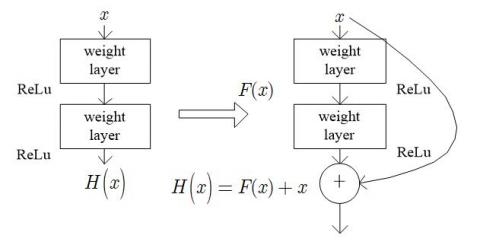Figure 1. Structure of a typical residual block

Google’s Inception-ResNet-v2 network  was selected to extract image features. The core component of the network is the Inception Architecture, which acquires different local sensory fields with 1*1, 3*3 and 5*5 kernels, and extracts and fuses features on multiple scales. In this paper, the Inception Architecture is combined with residual block into the Inception-ResNet-X module. The introduction of residual block prevents the degradation of network performance caused by multiple network layers (i.e. the deep depth of the network). As shown in Figure 2, the final network model was created by integrating 20 similar modules of the Inception-ResNet-v2 network. Two submodules of Inception-ResNet-v2 network are described in Figures 3 and 4, respectively.

## 2.jpg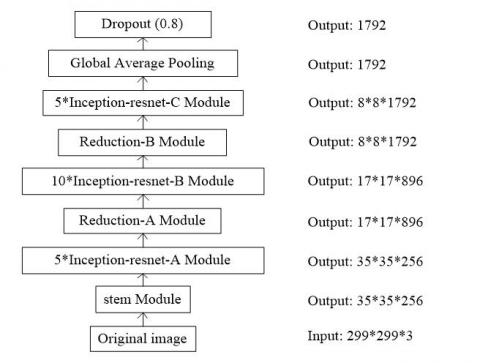Figure 2. Structure of Inception-ResNet-v2 network

## 3.jpg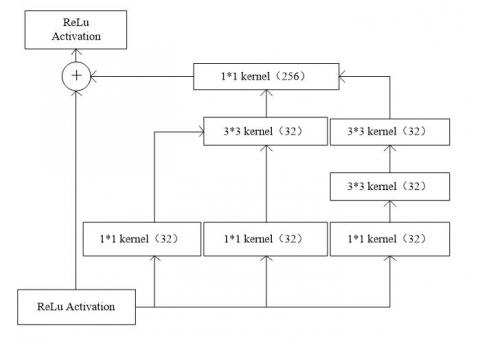Figure 3. Structure of Inception-ResNet-A module

## 4.jpgFigure 4. Reduction-A module

With the aid of the Inception-ResNet-v2 network, the image features were extracted in the following steps:

Step 1: The Inception-ResNet-v2 network was pre-trained with ImageNet dataset, and the pre-trained weight biases were saved for further use.

Step 2: The images of AICC training set were imported into the Inception-ResNet-v2 network for feature extraction, each imported image was normalized into the size of 229*229*3, the pre-trained weight biases were loaded into the network, and the softmax classification layer was removed.

Step 3: Based on the pre-trained weight biases, the Inception-ResNet-v2 network performed a series of convolution and pooling operations on the images, and finally the global average pooling layer output the 1,792-dimensional feature vector of each image.

Step 4: The image feature vectors and word feature vectors must be consistent in dimensions. Otherwise, the sentence generation model cannot be trained normally. Hence, the image feature mapping vectors were obtained by mapping the 1,792-dimensional feature vectors into the 512-dimensional word vector feature space through fully-connected operations. The mapping formula can be expressed as:

$y={{W}^{T}}\left( DCNN\left( I \right) \right)+b$    (1)

where y is a 512-dimensional feature vector obtained by fully-connected calculation; W is a 1,792*512-dimensional matrix; I is an image imported to the network; DCNN(I) is a 1,792-dimensional feature vector extracted by the network; b is a weight bias.

2.1 NNLM

The NNLM  is a language model constructed on the neural network. The model can represent word vectors through network optimization, and describe the word embedding with word distribution. The word embedding matrix offers an alternative to the sparse vector representation. In the NNLM, the n-gram construction is in the charge of the neural network. Figure 5 illustrates the structure of the NNLM.

The NNLM relies on its four-layer structure to predict the m-th word based on the first m-1 known words. The four layers are respectively the input layer, the embedding layer, the hidden layer and the output layer. The input layer receives the sparse word vectors; the embedding layer carries out word embedding of the input vectors and splices the word embedding vectors; the hidden layer executes the computing task; the output layer performs probability distribution of each words using the softmax classifier, and outputs a probability vector whose dimensionality equals the size of the dictionary.

## 5.jpg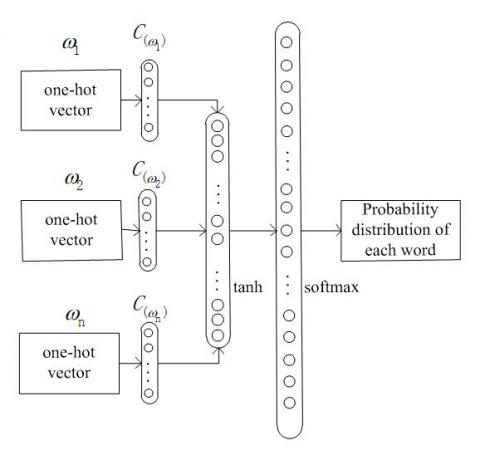Figure 5. The structure of the NNLM

2.2 Word encoding

Word encoding aims to generate a word embedding vector for each word in word frequency dictionary. The word embedding vectors have many advantages over one-hot vectors. With a relatively small dimensionality, the word embedding vectors overcome the sparsity problem of one-hot vectors. Since synonyms in word embedding matrix have similar distributions, the word vectors in word embedding matrix can express semantics, enhancing the relevance of sentences. Considering these advantages, our model relies on the NNLM to simulate one-hot vectors and replace them with word embedding vectors. The replacement reduces the training cost and improves the readability of the output sentences. The word encoding was implemented in the following steps:

Step 1: The set of Chinese alt texts was processed by the Chinese word segmentation tool Jieba . This set contains many low-frequency words, which should be filtered out. Otherwise, these words will dampen the convergence of the model, rather than promote the training effect.

Step 2: The words with frequency greater than 4 were selected and compiled into the word frequency dictionary, which serves as the index of word vectors.

Step 3: The <_START> and <_END> were defined to identify the start and end of each sentence. The two identifiers occupy one index bit. Through the above processing, the word frequency dictionary developed from the set of Chinese alt texts contains a total of 8,560 words.

Step 4: The words in the dictionary were subjected to one-hot encoding. The dimensionality of each one-hot vector equals the size of the dictionary. In each word vector, the bit number of 1 represents the index value of the word in the dictionary. Taking the word “person” for instance, the word vector should be encoded as [0 0 0 1 0…0 0]1*8560, because the index value is 3 in the dictionary.

Step 5: A word embedding matrix C8560*512 was randomly initialized. Based on trigram, a special case of the n-gram, three-word vectors w1*8560 were mapped through the matrix C8560*512 into the projection vectors [w*C]1*512. The three projection vectors were spliced into the output [w*C]1*1536 of the embedding layer. This embedding vector was sent to the hidden layer for nonlinear activation, and then transmitted to the output layer.

Step 6: In the NNLM, the number of output layer neurons equal the size of the word frequency dictionary. The nonlinearly activated embedding vector underwent probabilistic interpretation in the output layer by the softmax function. At the end of training, an 8,560-dimensional vector was outputted by the network. Each number in the vector represents the predicted probability of each word in the dictionary. Meanwhile, the parameters of the network model were updated, and the weight biases of the embedding layer were taken as the word embedding matrix.

Step 7: Word embedding and the NNLM were trained jointly. With the training of language model, the word embedding parameters were constantly updated until the model converged. Then, the trained word embedding matrix was obtained. During sentence generation, each one-hot vector searched for its corresponding word embedding vector in the word embedding matrix based on the index of word vectors. The word embedding vectors thus obtained were taken as inputs of the NNLM.

2.3 GRU

As a variant of the long-short term memory model (LSTM), the GRU is a recurrent neural network capable of solving long-term dependency problems [12, 13]. Compared with the LSTM, the GRU has a simple network structure, relatively few network parameters, good network performance and fast data training.

Based on the LSTM structure, the GRU neural network merges the cell state and hidden state, as well as the forget gate and the input gate. There are only two gates in the GRU, namely, the reset gate and the update gate. Like the LSTM, the GRU uses the two gates to screen and retain information. The screening and retainment are performed using a threshold in 0~1 set by sigmoid function. The reset gate controls how much the previous state of the hidden layer is forgotten. The value of the reset gate is negatively correlated with the amount of state information being forgotten. Meanwhile, the update gate controls how much the previous state of the hidden layer is retained in the current state of that layer. The value of the update gate is positively correlated with the amount of state information being retained. With the above structure, the GRU avoids the vanishing gradient problem, which often arises in backward derivation during the training of recurrent neural network (RNN), and prevents the loss of long-term memory in backward propagation. The structure of the GRU model is described in Figure 6, where ht-1 and ht are the previous and current states of the hidden layer, respectively.

## 6.jpg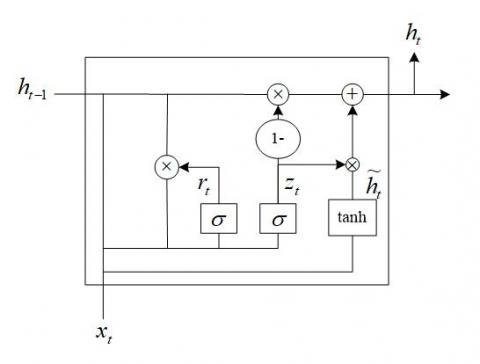Figure 6. Structure of the GRU model

The GRU network updates the hidden state through two gates. The specific steps are as follows:

Step 1: The reset gate rt controls how much the previous state of the hidden layer is forgotten. The forgetting degree helps to capture the short-term dependencies in the sequence data. The value of rt can be calculated by:

${{r}_{t}}=\sigma \left( {{W}_{r}}\cdot \left[ {{h}_{t-1}},{{x}_{t}} \right] \right)$      (2)

where σ() is the sigmoid function; Wr is the weight bias of the reset gate layer; ht-1 is the previous state of the hidden layer; xt is the current input.

Step 2: The update gate zt controls how much the previous state of the hidden layer is retained in the current state of that layer, i.e. the degree of impact of the previous state of the hidden layer on the current state of that layer. This impact degree helps to capture the long-term dependencies in the sequence data. The value of zt can be calculated by:

${{z}_{t}}=\sigma \left( {{W}_{z}}\cdot \left[ {{h}_{t-1}},{{x}_{t}} \right] \right)$     (3)

where σ() is the sigmoid function; Wz is the weight bias of the update gate layer; ht-1 is the previous state of the hidden layer; xt is the current input.

Step 3: The candidate state of the hidden layer refers to the state of the hidden layer to be retained at the current time. To determine the candidate state of the hidden layer, the previous state of the hidden layer is filtered at the reset gate through point multiplication between the value of the reset gate and the previous state of the hidden layer. The closeness of the reset gate value to zero describes how much the previous state is forgotten. In essence, the candidate state of the hidden layer is determined by multiplying the previous state of the hidden layer ht-1 and the current input with the weight bias, and then compressed into (-1, 1) with the tanh function. The candidate state of the hidden layer can be expressed as:

${{\tilde{h}}_{t}}=\tanh \left( W\cdot \left[ {{r}_{t}}*{{h}_{t-1}},{{x}_{t}} \right] \right)$    (4)

where W is the candidate weight bias; rt is the reset gate; ht-1 is the previous state of the hidden layer; xt is the current input.

Step 4: The current state of the hidden layer ht is the real output of the GRU network at the current time. To determine the current state of the hidden layer, the previous state of the hidden layer and the candidate state of the hidden layer are updated by the update gate. The closeness of the update gate value to one describes how much the previous state is retained. If the update gate equals one, then the previous state of the hidden layer will not attenuate over time and be retained fully to the current time. The current state of the hidden layer can be expressed as:

${{h}_{t}}=\left( 1-{{z}_{t}} \right)*{{h}_{t-1}}+{{z}_{t}}*{{\tilde{h}}_{t}}$      (5)

2.4 Sentence generation model

In this paper, the sentence generation model is created based on the double-layer GRU network, and used to predict words. The double-layer GRU network (Figure 7) was selected, for the model with more layers can learn deeper text features and acquire stronger fitting ability, making the sentences more accurate.

## 7.jpg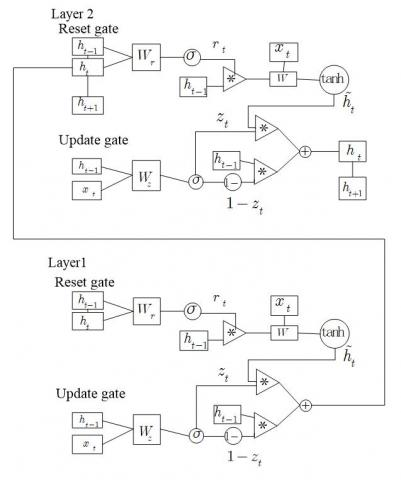Figure 7. Structure of double-layer GRU network

Layer 1 integrates image features with word embedding features, and inputs the results into Layer 2. According to the results from Layer 1, Layer 2 predicts and generates words by feature inference and decoding. The information flow in the model can be described as follows.

At t=0, Layer 1 receives (1) the image features obtained through feature mapping and (2) the word embedding features obtained through secondary encoding of encoded sparse words. Meanwhile, Layer 1 produces (1) the hidden layer input of Layer 1 at t=1 and (2) the actual input of Layer 2 at t=0.

At t=0, Layer 2 receives (1) the hidden layer output of Layer 1 and (2) the initial hidden layer value of Layer 2. Meanwhile, Layer 2 produces (1) the actual output of Layer 2 at t=0, and (2) the hidden layer input of Layer 2 at t=1.

The sentence generation model was constructed through the following steps:

Step 1: The features of the original image were extracted by the DCNN, and subjected to feature mapping. The resulting 512-dimensional feature vector P was taken as the input of Layer 1 of the GRU network.

Step 2: The string <_START> was added to a tagged Chinese sentence containing m words to identify the start of the sentence, and its bit was denoted as W0 (if the sentence has fewer than m words, the vacant positions should be filled up with zeros). The tagged sentence was subjected to word segmentation, and then converted into a list of index values of the words (e.g. [0, 1, 2, 5, 199, 0]). According to the index values in the list, the word embedding vector of each word in the sentence was looked for in the text feature mapping matrix W512*8560. In this way, the word embedding vectors of all the words in the sentence was determined as W1, W2, …, Wm (The feature space of the word embedding vector has 512 dimensions).

Step 3: At time t=0, the image feature vector P1*512 was inputted to Layer 1 to produce the hidden layer states h01 and h02. Among them, h01 was taken as the hidden layer input of Layer 1 at t=1, and h02 as the actual input of Layer 2 at t=0.

Step 4: At time t=1, the word embedding vector W0 (start identifier) was taken as the input to the input layer of Layer 1 at t=1. The reset gate rt obtained the reset threshold r11 by formula (2), while the update gate zt obtained the update threshold z11 by formula (3). The r11 controlled the forgetting degree of h01, and computed the candidate state of the hidden layeraccording to W0 and formula (4). The z11 controlled the retainment degree of h01 and h02, and calculated the hidden layer state h11.

Step 5: Taking the h11 as the actual input to the input layer of Layer 2 at t=1, the hidden layer state h12 of Layer 2 at t=1 was obtained through Steps 3 and 4, considering the hidden layer state h02 at t=0.

Step 6: Taking the h11 as the hidden layer input of Layer 1 at t=2, the hidden layer state h21 of Layer 1 at t=2 was obtained through Steps 3 and 4, considering the actual input W1 to the input layer of Layer 1 at t=2.

Step 7: The above steps were repeated at each time step until the last time step t (t=m). The final output of Layer 2 was thus obtained as ht2. In each time step, the hidden layer state hi2 of Layer 2 (the output of Layer 2 has 512 dimensions) was subjected to fully-connected calculation. The fully-connected layer has 8,560 neurons. In addition, any output from the fully-connected layer must go through probabilistic interpretation on the softmax layer. The softmax function can be expressed as:

$soft\max \left( x \right)=\frac{{{e}^{{{x}_{i}}}}}{\sum\nolimits_{j}{{{e}^{{{x}_{j}}}}}}$       (6)

where xi is the output of each neuron on the fully-connected layer; j is the total number of neurons on the fully-connected layer.

The softmax layer was added to ensure that the model outputs a probability vector, whose dimensionality is the size of the dictionary, at each time step. The vector reflects the probability that each of the 8,560 words is correct at the current moment. The probability vectors produced at all time steps were saved to construct the loss function.

Step 8: The tagged sentence was segmented into words, and converted to one-hot vectors, forming a sparse matrix Y of the size (m*n, 8,560). Only one bit in each row of the matrix is valued one, which corresponds to the index value of the word in the dictionary. All the other bits in the row are zeros. The model output P of Step 7 is also a (m*n, 8,560) matrix, in which each row specifies the probability values of all 8,560 words in the dictionary.

Step 9: The loss function was constructed based on the sparse matrix and the model output. Considering the specific tasks of the sentence generation model, the cross-entropy loss function was selected:

$L\left( {{y}_{i}},{{p}_{i}} \right)=-\sum\limits_{k=1}^{n\_batch}{\sum\limits_{i=0}^{m*n}{\sum\limits_{j=0}^{w\_count}{{{y}_{j}}\log \left( {{p}_{j}} \right)}}}$    (7)

where n_batch is the batch number of the training dataset; m is the sentence length; n is the mini-batch, i.e. the number of tagged sentences in a batch of data; w_count is the size of the dictionary (8,560); yj is the value at j-th bit of the word vector; pj is the probability value at the j-th bit of the model output vector.

Cross-entropy reflects the distance between the actual output and the expected output. The smaller the cross-entropy, the closer the two probability distributions. In this paper, the cross-entropy loss function is optimized such that the probability to correctly predict a word is close to one. In other words, the optimization goal is to maximize the probability that the index value and the word occupy the same bit.

Step 10: During model training, the network parameters were updated by time-based back-propagation algorithm . After the training, the original image was imported into the model to generate the alt text. The model firstly mapped the image features, and then took the image coding vector and the start identifier <_START> as the input of the double-layer GRU network at t=1. In light of the trained weight biases, the GRU network generated an 8,560-dimensional predicted word vector, and saved the word with the highest probability as the input at t=2. In this way, the network predicted and outputted a word at each time step, until reaching the end identifier <_END> or the preset length m. Finally, all generated words were combined into the Chinese alt text of the original image.

The structure of the sentence generation model is illustrated in Figure 8 below.

## 8.jpg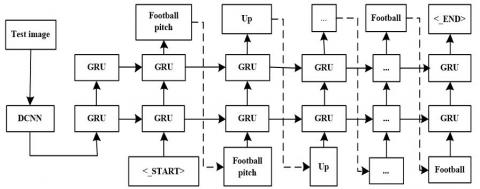Figure 8. The structure of the sentence generation model

3. Experiment and Results Analysis

3.1 Sample preparation and experimental environment

Our Chinese alt text writing model was designed on Ubuntu 17.10, written in Python, and constructed under TensorFlow 1.1.0. Once constructed, the model was trained and evaluated with the AICC Chinese alt text dataset. The training set was divided into a set of 210,000 images and a set of Chinese alt texts for these images. Each image has five alt texts. Figure 9 shows part of the training set.

To facilitate training, the data in the training set were converted into the TFRecord format that can be executed by TensorFlow. After conversion, each piece of data contains the number, binary code, storage path and name of the original image and a list of Chinese alt texts, which have undergone word segmentation.

In addition, the data in the AICC testing set were converted into the same format as those in the AICC training set, such that the model performance can be evaluated by the perplexity index. The AICC testing set consists of a set of 30,000 images and a set of 150,000 Chinese alt texts.

## 9a.png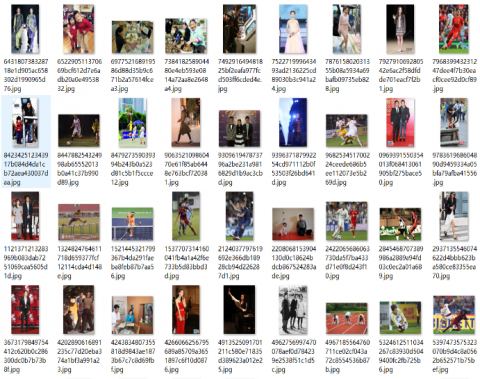(a) The set of images

## 9b.png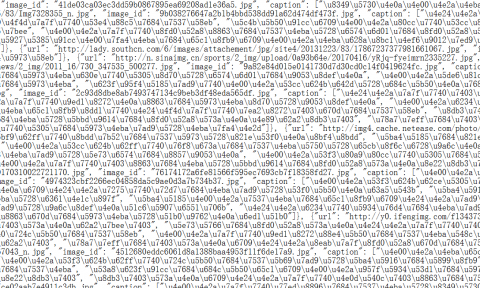(b)The set of Chinese alt texts

Figure 9. Part of the training set

3.2 Model training

The model was trained through forward propagation and backpropagation.

(1) Forward propagation

The TFRecord file was loaded into the memory to parse the image information. Then, the image data were imported in batches to the Incepton-ResNet-v2 network for feature extraction. Meanwhile, the dictionary of words describing the images was created, and then the word embedding vector was looked for in the word embedding matrix based on the index value. On this basis, the image feature vector and word embedding vector were inputted to the double-layer GRU network. Taking the image feature I at t=0 as the initialization coefficient of the hidden layer state, the words in the dictionary were embedded into matrix [W1, W2,...,Wm] and imported into the network step by step (t=1, 2,..., m). The network derived an 8,560-dimensional probability vector in each time step, until outputting the final feature vector at the m-th time step. The vector predicted in each time step was saved and spliced into an 8560*m-dimensional matrix. The matrix was outputted as the prediction result, making the end of the forward propagation.

(2) Backpropagation

During backpropagation, the gradient descent algorithm is often employed to update the network parameters. In the traditional batch gradient descent algorithm, the parameters are updated with all training data in each iteration. If applied to train deep learning models, this algorithm often faces problems like slow training and memory overflow. To solve the problems, the entire training set is usually segmented into subsets called mini-batches, before applying the algorithm for big data training of deep learning networks. The mini-batch-based training algorithm is known as the mini-batch gradient descent algorithm. This algorithm updates network parameters in the following way:

$\theta =\theta \text{-}\alpha \cdot \nabla J\left( \theta \right)$      (8)

where θ is the parameter to be updated; θ is the learning rate; J is a function about θ.

${{v}_{dw}}={{\beta }_{1}}{{v}_{dw}}+\left( 1-{{\beta }_{1}} \right)dw$      (9)

where vdw is the weighted moving average of gradient dw; β1=0.9 is a hyper-parameter.

${{s}_{dw}}={{\beta }_{2}}{{s}_{dw}}+\left( 1-{{\beta }_{2}} \right)d{{w}^{2}}$    (10)

where sdw is the weighted moving average of the gradient square dw2; β2=0.999 is a hyper-parameter.

$v_{dw}^{correct}=\frac{{{v}_{dw}}}{\left( 1-\beta _{1}^{t} \right)}$     (11)

where $v^{correct}_{dw}$ is the bias correction of the first moment estimation vdw; t is the number of iterations.

$s_{dw}^{correct}=\frac{{{s}_{dw}}}{\left( 1-\beta _{2}^{t} \right)}$   (12)

where $s^{correct}_{dw}$ is the bias correction of the second moment estimation sdw; t is the number of iterations.

The weight bias w can be updated by:

$w=w-\alpha \frac{v_{dw}^{correct}}{\sqrt{s_{dw}^{correct}}+\varepsilon }$   (13)

where ε=10-8 is a hyper-parameter to prevent the parameter update from being affected by insufficient bias correction.

The chain derivation of loss function was carried out using the predicted word vector and the actual word vector, and the model parameters were updated by Adam algorithm. To prevent over-fitting, the dropout mechanism was adopted for the fully-connected layer, randomly killing half of all neurons. The maximum number of iterations was set to 13,000. The iteration was terminated after reaching the set number or model convergence. The training curves of our model and the NIC model are shown in Figures 10 and 11, respectively. In the two figures, the y-axis represents the loss, the x-axis represents the number of iterations, the solid line indicates the loss after fitting, and the dotted line indicates the actual loss.

## 10.pngFigure 10. The training curve of our model

## 11.png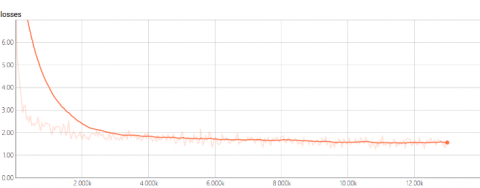Figure 11. The training curve of the NIC model

With the increase in the number of iterations, the loss of our model exhibited an obvious decline and the network parameters were updated. The iteration stopped after reaching the preset maximum number of iterations. It can be seen from Figures 10 and 11 that our model, using the dual-layer GRU network, converged faster than the NIC model, which adopts the LSTM network.

3.3 Objective evaluation indices

3.3.1 Perplexity

Perplexity is an index that measures the quality of the language model in natural language processing (NLP). Designed on the features of the language model, the index can be mathematically expressed as an exponent of cross-entropy:

$PPL={{e}^{-\frac{1}{m}\sum\limits_{i=1}^{m}{\log p\left( {{x}^{\left( i \right)}} \right)}}}$   (14)

where m is the number of sentences in the testing set; p(x(i)) is the probability of the i-th sentence. The greater the product of the probabilities p(x(i)) of all sentences x(i) in the testing set, the better the model performs on the testing set. The value of p(x(i)) can be obtained by:

$p\left( {{x}^{\left( i \right)}} \right)=\sum\limits_{j=1}^{n}{{{y}_{j}}\log {{p}_{j}}}$    (15)

where n is the number of words in each sentence; yj is the vector of the j-th tagged word; pj is the predicted probability of the j-th word.

Perplexity demonstrates the ability of the language model to predict a sentence. The greater the probability of the sentence, the smaller the Perplexity, and the more accurate the predicted sentence.

3.3.2 Bilingual evaluation understudy (BLEU)

The BLEU metric evaluates the machine translation based on n-word matching (the generated sentence and the example sentence are identical in any five consecutive words). The central idea behind the BLEU is that “the closer a machine translation is to a professional human translation, the better it is”. The evaluation criteria include fluency and grammatical correctness. The value of the BLEU index can be calculated by:

$B=BP\cdot \exp \left( \sum\limits_{i=1}^{N}{{{w}_{n}}\log {{P}_{n}}} \right)$      (16)

BP=\left\{ \begin{align} & 1\quad \quad \quad c>r \\ & {{e}^{1-r/c}}\quad \,\,c\le r \\\end{align} \right.      (17)

${{P}_{n}}=\frac{\sum\nolimits_{i}{n_{\min }^{i}}}{\sum\nolimits_{i}{count\left( {{w}_{i}} \right)}}$     (18)

where BP is the penalty factor; c is the length of the predicted sentence; r is the length of the correct sentence the closest to the length of the predicted sentence; N is the number of consecutive words that are identical between the correct sentence and the predicted sentence (the maximum value of N is usually set to 4); wn=1/N; Pn is the mean identical rate of words between the correct sentence and the predicted sentence.

3.3.3 Recall-oriented understudy for gisting evaluation (ROUGE)-L

ROUGE-L is a metric based on the longest common subsequence (LCS):

$ROUG{{E}_{L}}\left( {{c}_{i}},{{S}_{i}} \right)=\frac{\left( 1+{{\beta }^{2}} \right){{R}^{l}}{{P}^{l}}}{{{R}^{l}}+{{\beta }^{2}}{{P}_{l}}}$     (19)

${{R}_{l}}=\underset{j}{\mathop{\max }}\,\frac{l\left( {{c}_{i}},{{s}_{ij}} \right)}{\left| {{s}_{ij}} \right|}$      (20)

${{P}_{l}}=\underset{j}{\mathop{\max }}\,\frac{l\left( {{c}_{i}},{{s}_{ij}} \right)}{\left| {{c}_{i}} \right|}$     (21)

where β=Rl/Pl; |ci is the length of the predicted sentence; |sij| is the length of the reference sentence in the set of alt texts.

3.4 Model testing and evaluation

3.4.1 Model testing

The set of images in the AICC testing set was adopted to test our Chinese alt text writing model and the NIC model. The alt texts generated by the two models are recorded in Figure 12 below.

## 10a.jpg(a)

Ⅰ): There is a woman wearing a skirt performing on the stage.

Ⅱ): There are two people in costumes performing on the stage

## 10b.jpg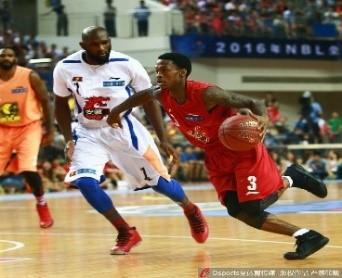(b)

Ⅰ): There are two men in jerseys fighting for possession in the court.

Ⅱ): There are three men in jerseys playing basketball in the court.

## 10c.jpg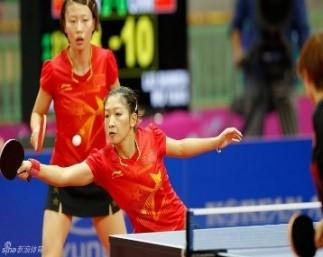(c)

Ⅰ): There are two people in sports clothes playing pingpong in the stadium.

Ⅱ): There are two people in sports clothes playing volleyball in the stadium.

## 10d.jpg(d)

Ⅰ): There is a man singing on the stage, holding the microphone in his right hand.

Ⅱ): There is a woman singing on the stage, holding the microphone in her right hand.

## 10e.jpg(e)

Ⅰ): There is a man wearing a hat working in the room.

Ⅱ): There is a man wearing a hat working in the kitchen.

## 10f.jpg(f)

Ⅰ): There is a woman singing, holding the microphone in her right hand, before two people on the stage.

Ⅱ): There is a woman singing on the stage, holding the microphone in her right hand.

Figure 12. The results of model testing

In Figure 12, the alt texts marked with I) were generated by our model, and those marked with II) were produced by the NIC model. As shown in Figure 12(a), our model outputted more accurate description of image content than the NIC model. From Figures 12(b)~(d), it is learned that our model could revise the mistakes in the alt texts. For example, there are “two” men fighting for possession in Figure 12(b), instead of “three”. It can be seen from Figure 12(e) that our model showed better accuracy than the NIC model in the derivation and summary of image content. Figure 12(f) indicates our model outperformed the NIC model in deriving and summing up the scenes that are difficult to describe.

In summary, our model can make intelligent inference on image content, identify the scenes, subjects, subject actions, and subject-object relations in the image, and describe the image content with a complete and coherent Chinese alt text.

3.4.2 Model evaluation

Our model, the NIC and the v2-LSTM were rated by objective evaluation indices of Perplexity, BLEU and ROUGE-L. The v2-LSTM was created by replacing the Inception-v3 in the NIC model with the Inception-ResNet-v2 of our model, and used to verify the feature extraction effect of the Inception-ResNet-v2.

The Perplexity scores of the three models were rated after 13,000 iterations on the AICC testing set. The results are listed in Table 1.

Table 1. The Perplexity scores of the three models

 Name of model Perplexity score Our model 4.922 v2-LSTM 5.026 NIC 5.044
The Perplexity score is negatively correlated with the quality of the language model. From Table 1, it can be seen that our model had a smaller Perplexity score than the other two models at the 13,000-th iterations on the AICC testing set. This means our model outperformed the contrastive models at the end of iterations.

Then, the BLEU index was adopted to evaluate the models based on simple sentences. The output sentences were compared with the tagged sentences of each test image, and then evaluated by the BLEU metric. The evaluation results are shown in Table 2 below.

Table 2. The BLEU values of the three models

 Name of model BLEU-1 BLEU-2 BLEU-3 BLEU-4 Our model 0.674 0.533 0.416 0.330 v2-LSTM 0.657 0.496 0.390 0.309 NIC 0.616 0.469 0.341 0.264
According to the results in Table 2, our model received higher BLEU-1, BLEU-2, BLEU-3 and BLEU-4 than the two contrastive models, leading the NIC by an average of 0.065 point. The comparison demonstrates the clear edge of our model over the NIC in describing the image content.

Finally, the three models were evaluated by the ROUGE-L index. The results are listed in Table 3 below.

Table 3. The ROUGE-L values of the three models

 Name of model ROUGE-L value Our model 0.635 v2-LSTM 0.593 NIC 0.555
Through experimental analysis and model testing, it can be seen that our model could identify the image content and describe it accurately with Chinese alt text. The results of objective evaluation manifest a clear advantage of our model over the other models. It is safe to say that our model can describe image content in Chinese more satisfactorily than NIC and other models.
4. Conclusions

This paper attempts to generate accurate and coherent Chinese alt texts for images. Drawing on the classic image captioning model NIC, the author designed a novel Chinese alt text writing model, coupling the DCNN model and deep GRU network. The NIC model was improved in two aspects, namely, image feature extraction and Chinese word encoding, and a new sentence generation model was created. On this basis, a high-performance alt text writing model was obtained. Through testing and objective evaluation, our model was proved as capable of generating Chinese alt texts for images, and outperform the NIC model in accuracy, coherence and readability of the generated sentences.

Acknowledgment

Project Supported by Natural Science Foundation of Heilongjiang Province (LH2019E058); University Nursing Program for Young Scholars with Creative Talents in Heilongjiang Province (UNPYSCT-2017091); Fundamental Research Fundation for Universities of Heilongjiang Province (LGYC2018JC027).

References

 Bernardi, R., Cakici, R., Elliott, D., Erdem, A. (2016). Automatic description generation from images: A survey of models, datasets, and evaluation measures. Journal of Artificial Intelligence Research, 55(1): 409-442. http//doi.org/10.1613/jair.4900

 Farhadi, A., Hejrati, M., Sadeghi, M.A., Young, P. (2010). Every picture tells a story: generating sentences from images. Lecture Notes in Computer Science, 21(10): 15-29. http//doi.org/10.1007/978-3-642-15561-1_2

 Karpathy, A., Li, F.F. (2015). Deep visual-semantic alignments for generating image descriptions. IEEE Computer Vision and Pattern Recognition, Boston, USA, 3128-3137. http//doi.org/10.1109/TPAMI.2016.2598339

 Xu, J., Gawes, E., Fernando, B., Tuytelaars, T. (2015). Guiding the long short-term memory model for image caption generation. IEEE International Conference on Computer Vision, Santiago, Chile, pp. 2407-2415. http//doi.org/10.1109/ICCV.2015.277

 Vinyals, O., Toshev, A., Bengio, S., Erhan, D. (2015). Show and tell: a neural image caption generator. IEEE Computer Vision and Pattern Recognition, Boston, USA, 3156-3164. http//doi.org/10.1109/CVPR.2015.7298935

 Krizhevsky, A., Sutskever, I., Hinton, G.E. (2012). Imagenet classification with deep convolutional neural networks. Neural Information Processing Systems, Lake Tahoe, USA, 1097-1105. http//doi.org/10.1145/3065386

 Yu, Y.W., Yin, G.F., Yin, Y., Du, L.Q. (2014). Radiographic image defect recognition method based on deep learning network. Chinese Journal of Scientific Instrument, 35(9): 2012-2019. http//doi.org/10.19650/j.cnki.cjsi.2014.09.012

 He, K., Zhang, X., Ren, S.Q., Sun, J. (2016). Deep residual learning for image recognition. IEEE Computer Vision and Pattern Recognition, Las Vegas, USA, 770-778. http//doi.org/10.1109/CVPR.2016.90

 Szegedy, C., Ioffe, S., Vanhoucke, V. (2016). Inception-V4, Inception-Resnet and the impact of residual connections on learning. AAAI Conference on Artificial Intelligence, Menlo Park, California, 4-12. arXiv preprint arXiv:1602.07261

 Bengio, Y., Schwenk, H., Senécal, J. (2003). Neural probabilistic language models. Journal of Machine Learning Research, 3(6): 1137-1155.

 Xie, J.B., Hou, Y.J., Kang, S.Q., Li, B.W., Zhang, X. (2018). Multi-feature fusion based on semantic understanding attention neural network for Chinese text categorization. Journal of Electronics & Information Technology, 2018(5). http//doi.org/10.11999/JEIT170815

 Huang, L., Du, C.S. (2017). Research on text classification based on recursive neural network. Journal of Beijing University of Chemical Technology (Natural Science Edition), 44(1): 98-104.  http//doi.org/CNKI: SUN: BJHY.0.2017-01-017

 Gers, F.A., Schmidhuber, J., Cummins, F. (2000). Learning to forget: Continual prediction with LSTM. Neural Computation, 12(10): 2451-2471. http//doi.org/10.1162/089976600300015015

 Werbos, P.J. (1990). Backpropagation through time: What it does and how to do it. Proceedings of the IEEE, 78(10): 1550-1560. http//doi.org/10.1109/5.58337

 Duchi, J., Hazan, E., Singer, Y. (2011). Adaptive sub gradient methods for online learning and stochastic optimization. Journal of Machine Learning Research, 12(7): 257-269. http//doi.org/10.1109/TNN.2011.2146788

 Kingma, D.P., Ba, J. (2015). Adam: a method for stochastic optimization. International Conference on Learning Representations, San Diego, USA, 1-13. arXiv preprint arXiv:1412.6980.